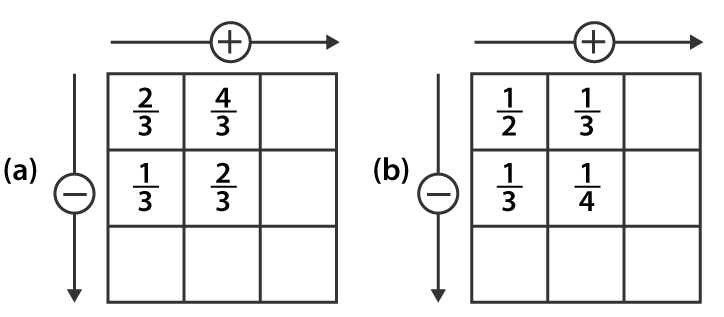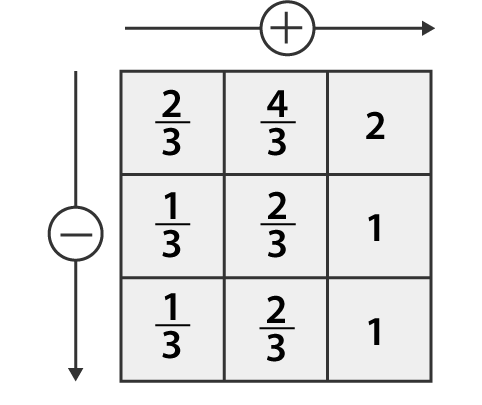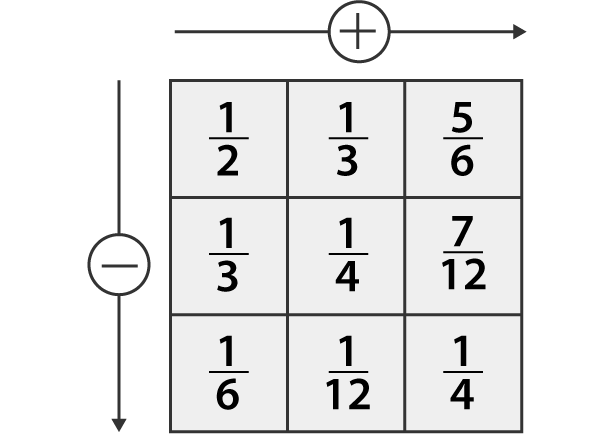# Complete the addition and subtraction box.

SolutionSolution:

(a) 2 / 3 + 4 / 3

= (2 + 4) / 3

= 6 / 3

= 2

1 / 3 + 2 / 3

= (1 + 2) / 3

= 3 / 3

= 1

2 / 3 – 1 / 3

= (2 – 1) / 3

= 1 / 3

4 / 3 – 2 / 3

= (4 – 2) / 3

= 2 / 3

1 / 3 + 2 / 3

= (1 + 2) / 3

= 3 / 3

= 1

Hence, the given box can be completed as shown below(b) 1 / 2 + 1 / 3

= [(1 × 3) + (1 × 2)] / 6

= (3 + 2) / 6

= 5 / 6

1 / 3 + 1 / 4

= [(1 × 4) + (1 × 3)] / 12

= (4 + 3) / 12

= 7 / 12

1 / 2 – 1 / 3

= [(1 × 3) – (1 × 2)] / 6

= (3 – 2) / 6

= 1 / 6

1 / 3 – 1 / 4

= [(1 × 4) – (1 ×3)] / 12

= (4 – 3) / 12

= 1 / 12

1 / 6 + 1 / 12

= [(1 × 2) + 1] / 12

= (2 + 1) / 12

= 3 / 12

= 1 / 4

Hence, the given box can be completed as shown below(0)(0)